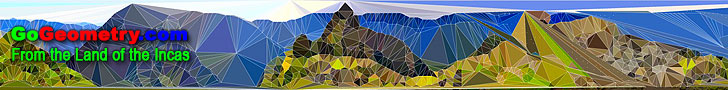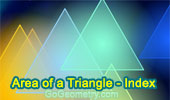# Welcome to GoGeometry: Recent additions (Page 3), Online LearningGo Geometry from the Land of the Incas - Recent Additions (Page 3 of 50)Geometry Problem 960. Quadrilateral, Trisection, Sides, Congruence, Similarity, Triangle. Geometry Problem 959. Triangle, Sides Ratio 4:1, Inradius, Exradius, Cevian, Mean Proportional, Geometric Mean, Metric Relations. Geometry Problem 958. Isosceles Triangle, Altitude, 45 Degrees, Perpendicular, Angle Bisector, Metric Relations. Geometry Problem 957. Equilateral Triangle, Inscribed Circle, Incircle, Circumscribed Circle, Circumcircle, Area, Circular Segment. Geometry Problem 956. Two Equilateral Triangles, Center, Collinear Points, Midpoint, Right Triangle, 30, 60, 90 Degrees. Geometry Problem 955. Quadrilateral, Triangle, Diagonals, Triangle, Angles, 30, 40, 60, 70 Degrees. Geometry Problem 954. Intersecting Circles, Secant, Common Chord, Cyclic Quadrilateral, Angle Bisector. Geometry Problem 953. Intersecting Circles, Secant, Common Chord, Cyclic Quadrilateral, Perpendicular. Geometry Problem 952. Triangle, Incenter, Circumcenter, Three Equal Circles, Tangent Line, Tangent Circles, Collinear Points. Geometry Problem 951. Intersecting Circles, Chord, Diameter, Parallel, Cyclic Quadrilateral, Concyclic Points. Geometry Problem 950. Intersecting Circles, Secant, Cyclic Quadrilateral, Concyclic Points. Geometry Problem 949. Intersecting Circles, Secant, Cyclic Quadrilateral, Concyclic Points. Math Quote: Galileo Galilei. Word cloud. Geometry Problem 948. Intersecting Circles, Secant, Cyclic Quadrilateral, Concyclic Points. Puzzle: Euler Line and Nine Point Center HTML5 canvas for iPad, Nexus and touch Devices. Geometry Problem 947. Triangle, Circumcircle, Angle Bisector, Chord, Arc, Parallel. Math Quote: Aristotle. Word cloud. Geometry Problem 946. Triangle, Quadrilateral, Area, Diagonal, Midpoint, Parallel. Geometry Problem 945. Triangle, Concurrent Lines, Cevians, Perpendicular, Angle Bisector. Geometry Problem 944. Triangle, Orthocenter, Altitude, Perpendicular, 90 Degrees, Parallel Lines. Go to Page: Previous | 1 | 2 | 3 | 4 | 5 | 6 | 7 | 8 | 9 | 10 | 11 | 12 | 13 | 14 | 15 | 16 | 17 | 18 | 19 | 20 | 21 | 22 | 23 | 24 | 25 | 26 | 27 | 28 | 29 | 30 | 31 | 32 | 33 | 34 | 35 | 36 | 37 | 38 | 39 | 40 | 41 | 42 | 43 | 44 | 45 | 46 | 47 | 48 | 49 | 50 | Next

 Home | GoGeometry Education | Geometry | Search | Email Last updated Aug 25, 2014